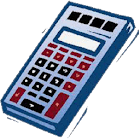Scientific Calculator

All Android applications categories

All Android games categories# Scientific Calculator

by: 6 9.4

9.4 Users
rating

## Screenshots

Description

This is the most easy-to-use and advanced scientific calculators out there with features including:
log, sin, cos, tan, clear, sqrt, asin, acos, atan, pi, ln, ee, parenthesis, division, squares, multiplication, exponents, subtraction, power of ten, addition, del, modulus (%), and the ability to evaluate a whole expression.

Tags: calculator , scientific calculator , scientific calculator code in , calculator code , scientific calculator code , scientific calculator codes , calculator quellcode , scientific calculator in code , code calculator

from 6 reviews

"Awesome"

9.4